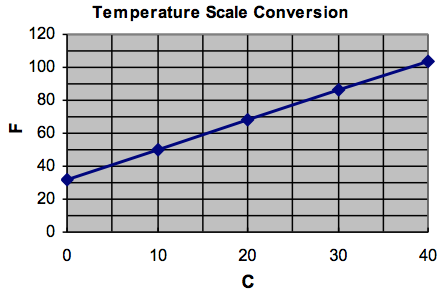$$\newcommand{\id}{\mathrm{id}}$$ $$\newcommand{\Span}{\mathrm{span}}$$ $$\newcommand{\kernel}{\mathrm{null}\,}$$ $$\newcommand{\range}{\mathrm{range}\,}$$ $$\newcommand{\RealPart}{\mathrm{Re}}$$ $$\newcommand{\ImaginaryPart}{\mathrm{Im}}$$ $$\newcommand{\Argument}{\mathrm{Arg}}$$ $$\newcommand{\norm}{\| #1 \|}$$ $$\newcommand{\inner}{\langle #1, #2 \rangle}$$ $$\newcommand{\Span}{\mathrm{span}}$$

# 28.1: D1.01: Introduction

$$\newcommand{\vecs}{\overset { \rightharpoonup} {\mathbf{#1}} }$$ $$\newcommand{\vecd}{\overset{-\!-\!\rightharpoonup}{\vphantom{a}\smash {#1}}}$$$$\newcommand{\id}{\mathrm{id}}$$ $$\newcommand{\Span}{\mathrm{span}}$$ $$\newcommand{\kernel}{\mathrm{null}\,}$$ $$\newcommand{\range}{\mathrm{range}\,}$$ $$\newcommand{\RealPart}{\mathrm{Re}}$$ $$\newcommand{\ImaginaryPart}{\mathrm{Im}}$$ $$\newcommand{\Argument}{\mathrm{Arg}}$$ $$\newcommand{\norm}{\| #1 \|}$$ $$\newcommand{\inner}{\langle #1, #2 \rangle}$$ $$\newcommand{\Span}{\mathrm{span}}$$ $$\newcommand{\id}{\mathrm{id}}$$ $$\newcommand{\Span}{\mathrm{span}}$$ $$\newcommand{\kernel}{\mathrm{null}\,}$$ $$\newcommand{\range}{\mathrm{range}\,}$$ $$\newcommand{\RealPart}{\mathrm{Re}}$$ $$\newcommand{\ImaginaryPart}{\mathrm{Im}}$$ $$\newcommand{\Argument}{\mathrm{Arg}}$$ $$\newcommand{\norm}{\| #1 \|}$$ $$\newcommand{\inner}{\langle #1, #2 \rangle}$$ $$\newcommand{\Span}{\mathrm{span}}$$

### Objectives

1. Distinguish between input values and output values in formulas.
2. Use the order of operations correctly evaluating formulas.
3. Choose an appropriate scale for each axis and graph the formula by hand.
4. Use the graph to find what input value will give a particular output value.
5. Use numerical methods to check that answer and to refine it to get a somewhat more accurate answer. (After learning to graph using a spreadsheet.)
6. Use formulas with several different variables as input.
7. Understand the use of subscripts in formulas.

Many formulas are used in geometry and other applications. It is important to be able to correctly plug in values and compute the result.   In this course we will also learn to graph some of these formulas so that we can investigate the patterns more fully.

Definition: Usually when people refer to an equation as a formula it has the output variable alone on one side of the equation. When the equation is written in that form, it is easy to evaluate the output variable at different input values and to easily graph the formula. When we use the word “formula” in this course, we will always mean that the output variable is alone on one side of the equation.

### Example 1

Example 1. In Canada and Mexico, weather reports report temperature using the Centigrade (or Celsius) scale.   In the US, temperature is reported using the Fahrenheit scale.   To convert temperature C to temperature F, we use the formula$F=\frac{9}{5}C+32$.   When the temperature C is 23°, what is the temperature F?\begin{align}&F=\frac{9}{5}C+32\\&F=\frac{9}{5}(23)+32\\&F=41.4+32\\&F=73.4\\\end{align}

Thus the temperature F is 73.4°.

Notice that we used the correct order of operations here, performing the multiplication before the addition.

Here we call the 23° the input value and the 73.4° the output value.  (In algebra class, we call the set of input values the “domain” and the set of output values the “range”.)

When we graph a formula, we will put the input value on the horizontal axis, sometimes called the x-axis, and the output value on the vertical axis, sometimes called the y-axis. We can use different letters for the variables besides x and y, but when we think of the formula graphically, we must be clear about which variable plays the role of x and which plays the role of y. (You should be able to do these graphs by hand as well as with a spreadsheet.)

Formula input and output
C F
23 73.4
0 32
10 50
20 68
30 86
40 104